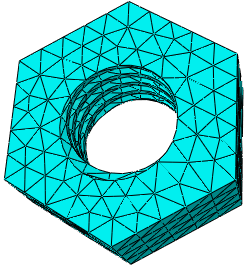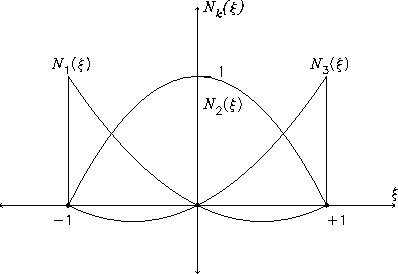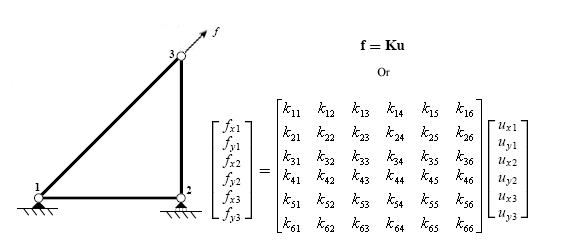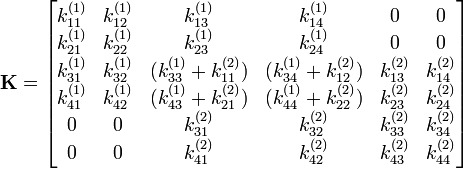﻿ Finite Element Method, a non-technical introduction

# Finite Element Method

## What is Finite Element Method (FEM)?

FEM is a comutational method for approximate solution of engineering and physical problems

### Why approximate solution?

Simply because general and exact solutions only exist for simple geometrical shapes and material behaviours while real world applications have complex physical shapes and properties

## Applications of FEM

• Mechanics: Solids, Fluids and Thermo-mechanics

• Civil, Structures and Geotechnics

• Biomechanics

• Electromagnetics

• Finance

## How it works? (I)

### Meshing# →The geometric shapes of the objects (domain of the problem) is divided into a FINITE number of small and well-defined ELEMENTs.

Elements will have EDGES (sides) and NODES (vertices)

## How it works? (II)

### Shape Function is DefinedA relation to relate the quantities inside the element to those quantities at the nodes are defined (SHAPE FUNCTION)

## How it works? (III)

### Deriving Constitutive Matrices of Elements• This local (e.g. stiffness) matrix defines the physical behaviour of its ELEMENT

• It relates the involving quantities (e.g. displacement and loads) to those values at the nodes of the elements

## How it works? (IV)

### Assembling Global Matrix• Element matrices will be assembled (if time is not involved) into a global matrix for all the elements

• Transformation of coordinates from local to global is used for assembling

## How it works? (V)

### Applying Boundary Conditions• Boundary Conditions (BCs) are applied at nodes

• Global stiffness matrix is modified accordingly

## How it works? (VI)

### Solving Global Matrix Equation##### Global Stiffness × unkowns = Loads
• The global matrix equation is solved using comutational linear algebra (libraries) giving values at the nodes

• Shape functions are used to determine values throughout elements

• Convergence

• Stability

• Efficiency